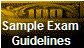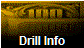Find It: Select a linkCourses PageFin 321Fin 321 Exam1 ReviewSample ExamsDrills IntroductionDrillsPassword RequestFin 425Data Fin 425 Cases Finance 425 TeamsFin 423Fin 423 dataFin 423 TeamsFin 423 Grower SpreadsheetCash BudgetingFin 423 Final ExamFin 323Fin 323 ProjectFin 323 Homework ProblemsOffice Hours and LocationMap to OfficeFeedbackFAQServices, Links etc.SDSU LinksDownload FilesFinancial sitesForecasting sitesEconomic sitesWhat's NewSiteMapGS 100Time ManagementInstructorTutorialsFin 323 GradesHomework ProblemsSearch This Site The WebFinance 321 Managerial Economics Exam I Topic Outline This is an outline of the topics for Exam 1, covering chapters 1-5. This is intended as an example outline. For the remaining exams you should outline the topics yourself. An outline helps you understand which topics are important and how they relate. Another source of an outline is in the front of the text (it's the table of contents).Outline Of Topics for Exam I  Managerial Economics Economics is? Scarce and Alternatives Resource Allocation Process Objectives of the Firm Wealth Maximization Agency Problems of Modern Corporations Marginalism Classical Optimization (Unconstrained) Second Order Conditions Constrained Optimization Two types of techniques Optimization Techniques Huristics Programming Solutions Economic Decisions and Uncertainty Risk Aversion Corporate Vs. Individuals Risk and Return Expected Utility Expected Value Why Insurance? Demand and Supply Theory of Demand Demand Schedule (in various forms) Total Revenue Where do Demand Curves Come From? Indifference Curves The Budget Constraint Consumer Choice Consumer Equilibrium The Effect of Price Changes Income and Substitution Effects The Demand Curve Shifting Demand Curves Other factors Shifts are Caused by? Market Demand Curve Elasticity Ways to Measure Price Elasticity Continuous Case ARC Elasticity Point Elasticity Total Revenue & Elasticity Elasticity and Marginal Revenue Market and Firm Demand Elasticity Income Elasticity Engel's Curves Cross Elasticity Other Elasticities Regression Analysis Uses for Simple Regression Assumptions of Regression Analysis Estimating the Regression Coefficients Regression Features of Spreadsheets Uses for Regression Equations Expectations for Model Results Does everything look Ok? Correlation Analysis Partitioning the Variance Analysis of Variance Calculating Correlation Values The T-Test The F-Test Using Spreadsheet Output Interpreting The Output Multiple Regression Difficulty with Regression-Data Patterns Autocorrelation Difficulty with Regression - Model Design Specification Problems Multicollinearity Problems Simultaneous Observations Non Linear Relationships Transforming Data Try a transformed plot Converting the Equation: Advantages and Disadvantages of Multiplicative Forms of Estimating the Demand Curve A good use for this outline is to look at the topics and see what you can say about them. For example: Can you define the terms? Can you describe how the concept is used? Can you think of an application? Can you find a problem in the appropriate chapter and solve it without help? Can you come up with a good question relating to the topic? Can you explain the idea and its use to someone else? In studying for the exam try to avoid memorization. If your memory is like mine you will forget just at the time you need it most--during an exam. Instead try to understand the process, rather than trying to remember the result. For example one of the concepts you are responsible for is elasticity. You could choose to memorize all of the possible elasticity forms and types of elasticity. A much better way would be to remember the concept of elasticity, simply a percentage change in one variable verses a percentage change in another variable. You can write that out in words, convert the words to symbols and then you can then convert that idea into various ways to define and manipulate a ratio of percentages as you need it. Thus if the question concerns Income elasticity (or any elasticity) you know that this is (written as a formula): (%Change in Quantity)/(%Change in Income) =( %Q)/(%Y )= (Q/Q)/(Y/Y). You realize that perhaps the best way to calculate percentages is over the average numbers thus you use: (Average %Q)/(Average%Y). You might choose to convert to a very small percentage change by using the form: (Q/Q)/(Y/Y) and changing to: (Q/Y)(Q/Y) and then change the 's to very small amounts which would result in the derivative form: (dQ/dY)(Q/Y). If you try to remember only this final formula and you forget--you're stuck. In other words, if you are going to add, learn the process of adding rather than trying to remember the answer to all possible addition problems.[Home] [Courses] [Instructor] [Services] [What's New] [SiteMap]Please see the FeedBack page with questions or comments. © Copyright 2007 Pieter A. Vandenberg. All rights reserved.